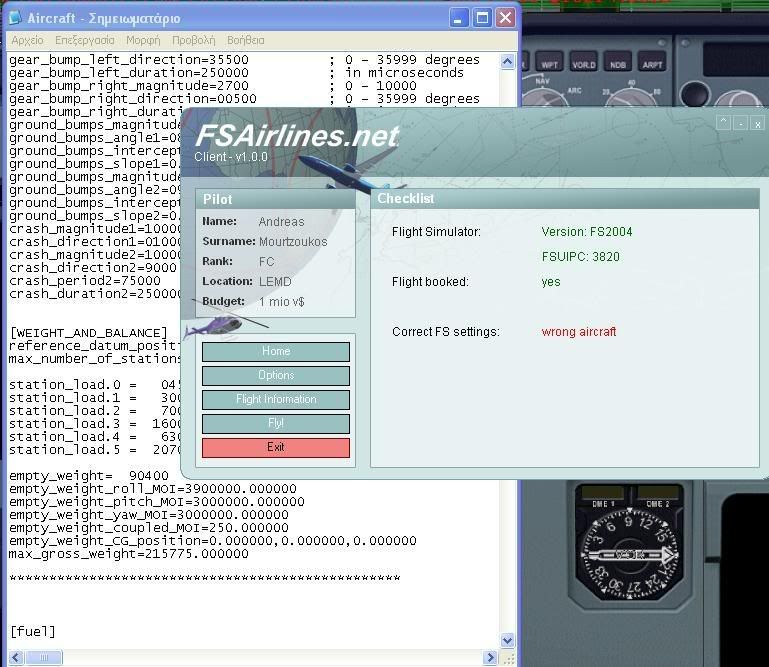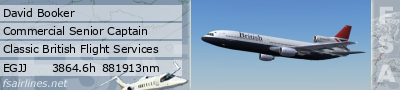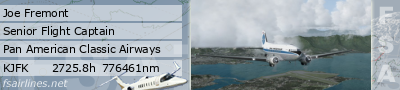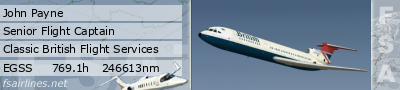## Wrong aircraft type

Please report Bugs and Problems here
Dave seventhree

### Re: Wrong aircraft type

ARK14 uk Aviator CEO Inst Glasgow Sep 20, 12:44 booked a 738 egpf to egnt.. opened fsx with a 738 fsx default, cient shows wrong aircraft.

Konny
FSAirlines Developer
Posts: 1564
Joined: Sun Sep 25, 2005 10:40 am
Location: Munich, Germany
Contact:

### Re: Wrong aircraft type

just had a look at the boeing specs for the 737-800:
http://www.boeing.com/commercial/airpor ... 37sec2.pdf

looks like there are quite different values for the w/wo winglets version. We had the one WITH winglets in our database, so I just changed that to refer to the without-winglets version. The client should detect both now.andymourtz
Ticket Agent
Posts: 3
Joined: Sat Sep 20, 2008 1:23 pm

### Re: Wrong aircraft type

Can anyone say something about Airbuses?
I booked a flight with a A320 but ''wrong aircraft'' was appeared

andymourtz
Ticket Agent
Posts: 3
Joined: Sat Sep 20, 2008 1:23 pm

### Re: Wrong aircraft typehere is my flight.I also included aircraft.cfg of my A320 Just Flight and the message i get.

vaccaro
Captain
Posts: 488
Joined: Sat Nov 25, 2006 12:03 pm

### Re: Wrong aircraft type

I am currently flying Project Airbus A320, all normal.
Might be a bug of that sh**ty PSS bus.

Konny
FSAirlines Developer
Posts: 1564
Joined: Sun Sep 25, 2005 10:40 am
Location: Munich, Germany
Contact:

### Re: Wrong aircraft type

hm, your max_gross_weight value is way of what it should be (about 170.000). An Addon like the PSS Airbus should be more accurate. You might change the value manually. 200.000 should be enough to be still within the toleranceandymourtz
Ticket Agent
Posts: 3
Joined: Sat Sep 20, 2008 1:23 pm

### Re: Wrong aircraft type

with the gross wheight in 200000 is now ok.I'll post again if there is any other problem in any other Airbus
Thanksrampas

### Re: Wrong aircraft type

I try to start to fly but new client writes me "WRONG AIRCRAFT"
I try to use Ilyushin 96 (IL96). Could you help me what should I do?

Herewith IL96 config:

[fltsim.0]
title=AEROFLOT IL-96
sim=il96
model=96
panel=96
sound=
texture=afl96
kb_checklists=
kb_reference=il96_ref
atc_id=RA96101
atc_airline=1
atc_flight_number=96005
atc_heavy=1
ui_manufacturer=Ilyushin
ui_type=Ilyushin Il-96
ui_variation=Aeroflot RA-96005
description=Visual model by D.Samborski, Panel by S.Golosov, Textures by I.Borodin, FDE from S.Dubovitsky FS2004.

[General]
atc_type=Ilyushin
atc_model=IL-96
editable=1
performance=Length:59 m\nWing span: 48 m\nWeight: 208 000 kg (MTOW)\nPower: Kuznetsov NK-86 x4\nPassengers: 350\nRange: 5000 km (2600 nm)

[WEIGHT_AND_BALANCE]
//reference_datum_position=-4.000, 0.000, -1.000
reference_datum_position=-4.000, 0.000, -1.000
empty_weight_CG_position=-2.740, 0.000, 0.000
max_number_of_stations=50
station_load.0=70000.0,0.000000,0.000000,0.000000, 320 passengers and 5 crew members
empty_weight=244600.000
empty_weight_roll_MOI=20037234.000
empty_weight_pitch_MOI=10000000.000
empty_weight_yaw_MOI=10022144.000
empty_weight_coupled_MOI=0.000
max_gross_weight=463000.000
CG_forward_limit=0.000
CG_aft_limit=1.000

[flight_tuning]
cruise_lift_scalar=1.000
parasite_drag_scalar=1.000
induced_drag_scalar=1.000
elevator_effectiveness=1.000
aileron_effectiveness=1.000
rudder_effectiveness=1.000
pitch_stability=1.000
roll_stability=1.000
yaw_stability=1.200
elevator_trim_effectiveness=1.000
aileron_trim_effectiveness=1.000
rudder_trim_effectiveness=1.000

[GeneralEngineData]
engine_type=1
Engine.0=-21.000, -57.167, -4.533
Engine.1=-2.500, -33.833, -6.500
Engine.2=-21.000, 57.167, -4.533
Engine.3=-2.500, 33.833, -6.500
fuel_flow_scalar=2.20
min_throttle_limit=-0.299988

[TurbineEngineData]
fuel_flow_gain=0.0020
inlet_area=19.60
rated_N2_rpm=7400.000
static_thrust=29160.000
afterburner_available=0
reverser_available=1

[jet_engine]
thrust_scalar=1.000

[electrical]
max_battery_voltage=28.0
max_generator_alternator_amps=40.0
flap_motor=0, 5 , 17.0
gear_motor=0, 0.5 , 17.0
autopilot=1, 0.5 , 17.0
avionics_bus=0, 1.2, 17.0
avionics=1, 0.2 , 17.0
pitot_heat=0, 0.2 , 17.0
marker_beacon=1, 0.5 , 17.0
gear_warning=0, 0.2 , 17.0
fuel_pump=0, 0.5 , 17.0
hydraulic_pump=0, 0.3, 17.0
starter1=0, 2.0, 17.0
starter2=0, 2.0, 17.0
starter3=0, 2.0, 17.0
starter4=0, 2.0, 17.0
light_nav=0, 2.5 , 17.0
light_beacon=0, 2.5 , 17.0
light_landing=0, 10.5 , 17.0
light_taxi=0, 5.5 , 17.0
light_strobe=0, 5.5 , 17.0
light_panel=0, 1.5 , 17.0
light_cabin = 0, 5 , 17.0

[contact_points]

//0 Class <0=none,1=wheel, 2=scrape, 3=float>
//1 Longitudinal Position (feet)
//2 Lateral Position (feet)
//3 Vertical Position (feet)
//4 Impact Damage Threshold (Feet Per Minute)
//5 Brake Map (0=None, 1=Left, 2=Right)
//7 Steer Angle (degrees)
//8 Static Compression (feet) (0 if rigid)
//9 Max/Static Compression Ratio
//10 Damping Ratio (0=Undamped, 1=Critically Damped)
//11 Extension Time (seconds)
//12 Retraction Time (seconds)
//13 Sound Type
//14 Airspeed limit for retraction (KIAS)
//15 Airspeed that gear gets damage at (KIAS)
// 0 1 2 3 4 5 6 7 8 9 10 11 12 13 14 15
point.0=1.000, 63.617, 0.000, -15.65, 1574.803, 0.000, 2.100, 60.000, 1.200, 1.200, 1.500, 9.000, 8.000, 0.000, 0.000, 0.000
point.1=1.000, -8.583, -16.583, -15.00, 1574.803, 1.000, 2.106, 0.000, 1.000, 0.800, 1.600, 10.000, 8.000, 2.000, 230.000, 1202.542
point.2=1.000, -8.583, 16.583, -15.00, 1574.803, 2.000, 2.106, 0.000, 1.000, 0.800, 1.600, 10.000, 8.000, 3.000, 230.000, 1202.542
//point.3=1.000, -12.5, 0.000, -18.00, 1574.803, 0.000, 2.106, 0.000, 4.200, 0.200, 0.600, 10.000, 8.000, 2.000, 230.000, 1202.542
//point.4=3.000, -32.000, -82.833, 5.250, 1574.803, 0.000, 0.000, 0.000, 0.000, 0.000, 0.000, 0.000, 0.000, 5.000, 0.000, 0.000
//point.5=4.000, -32.000, 82.833, 5.250, 1574.803, 0.000, 0.000, 0.000, 0.000, 0.000, 0.000, 0.000, 0.000, 6.000, 0.000, 0.000
//point.6=5.000, -62.083, 0.000, -2.833, 1574.803, 0.000, 0.000, 0.000, 0.000, 0.000, 0.000, 0.000, 0.000, 9.000, 0.000, 0.000
//point.7=6.000, 64.917, 0.000, -2.833, 1574.803, 0.000, 0.000, 0.000, 0.000, 0.000, 0.000, 0.000, 0.000, 4.000, 0.000, 0.000
//point.8=7.000, -30.90, 5.250, -7.300, 1600, 0, 0.00, 50.0, 0.0, 0.0, 1.00, 1.1, 1.5, 0, 0.0, 0.0
point.3= 5, -30.9, -5.25, -7.30,1600, 0, 0.00, 50.0, 0.0, 0.0, 1.00, 8.0, 8.2, 0, 0.0, 0.0

static_pitch=0.000
static_cg_height=16.000 // 16
gear_system_type=1

[Views]
eyepoint=83.34,-1.499446,7.04

Radio Type = availiable, standby frequency, has glide slope
Audio.1 = 1
Com.1 = 1, 0
Com.2 = 1, 0
Nav.1 = 1, 0, 1
Nav.2 = 1, 0, 0
Transponder.1 = 1
Marker.1 = 1

[LIGHTS]
//Types: 1=beacon, 2=strobe, 3=navigation, 4=cockpit, 5=landing
light.0=2, -34.00, 0.00, -8.50, fx_beaconb ,
light.1=1, -97.17, 0.00, 39.53, fx_beaconh ,
light.2=4, 90.1, 0.00, 11.45, fx_vclight ,
light.3=3, -54.36, 0.00, 4.70, fx_navwhih ,
//light.3=3, 63.617, 6.5, -15.033, fx_navredh ,
//light.4=3, -16.583, 20.583, -13.00, fx_navgreh ,
//light.5=3, -19.5, 3.000, -13.00, fx_navgreh ,

[keyboard_response]
elevator=150.000000,250.000000
aileron=150.000000,250.000000
rudder=150.000000,250.000000

[direction_indicators]
direction_indicator.0=3,0

[pitot_static]
vertical_speed_time_constant=2

[exits]
number_of_exits=2
exit_rate.0=0.4
exit_rate.1=0.6

[EFFECTS]
wake=fx_wake
water=fx_spray
dirt=fx_tchdrt
concrete=fx_sparks
touchdown=fx_154, 1

[autopilot]
autopilot_available = 1
flight_director_available = 1
default_vertical_speed = 1800.0
autothrottle_available = 1
autothrottle_arming_required = 1
autothrottle_max_rpm = 90
autothrottle_takeoff_ga = 1
pitch_takeoff_ga = 8;
max_pitch=10.0
max_pitch_acceleration=1.0
max_pitch_velocity_lo_alt=2.0
max_pitch_velocity_hi_alt=1.5
max_pitch_velocity_lo_alt_breakpoint=20000.0
max_pitch_velocity_hi_alt_breakpoint=28000.0
max_bank=25.0
max_bank_acceleration=1.8
max_bank_velocity=3.00
max_throttle_rate=0.10
nav_proportional_control=16.00
nav_integrator_control=0.17
nav_derivative_control=0.00
nav_integrator_boundary=2.50
nav_derivative_boundary=0.00
gs_proportional_control = 18.0
gs_integrator_control = 0.33
gs_derivative_control = 0.00
gs_integrator_boundary = 0.70
gs_derivative_boundary = 0.00
yaw_damper_gain = 1.0

//[autopilot]
//autopilot_available=1
//direction_indicator=1
//flight_director_available=1
//default_vertical_speed=1000
//autothrottle_available=1
//autothrottle_arming_required=1
//autothrottle_max_rpm = 100
//autothrottle_takeoff_ga=1
//pitch_takeoff_ga = 8;
//max_pitch=10.000000
//max_pitch_acceleration=0.600000
//max_pitch_velocity_lo_alt=1.000000
//max_pitch_velocity_hi_alt=0.600000
//max_pitch_velocity_lo_alt_breakpoint=20000.000000
//max_pitch_velocity_hi_alt_breakpoint=28000.000000
//max_bank=24.000000
//max_bank_acceleration=3.800000
//max_bank_velocity=4.000000
//max_throttle_rate=0.100000
//nav_proportional_control=9.000000
//nav_integrator_control=0.250000
//nav_derivative_control=0.000000
//nav_integrator_boundary=2.500000
//nav_derivative_boundary=0.000000
//gs_proportional_control=9.520000
//gs_integrator_control=0.260000
//gs_derivative_control=0.000000
//gs_integrator_boundary=0.700000
//gs_derivative_boundary=0.000000
//yaw_damper_gain=0.000000

[fuel]
//Center1=0.000, 0.000, 0.000, 10816.000, 0.000
//LeftMain=0.000, 0.000, 0.000, 5104.000, 0.000
//LeftAux=0.000, -63.000, 0.000, 4268.000, 0.000
//RightMain=0.000, 0.000, 0.000, 5104.000, 0.000
//RightAux=0.000, 63.000, 0.000, 4268.000, 0.000
Center1=0.000, 0.000, 0.000, 22000.000, 0.000
fuel_type=2.000000
number_of_tank_selectors=1
electric_pump=0

[airplane_geometry]
wing_area=4000.000 //3551.000
wing_span=257.667 // 157
wing_root_chord=24.830
wing_dihedral=6.476
wing_incidence=3.000
wing_twist=-4.000
oswald_efficiency_factor=0.720
wing_winglets_flag=0
wing_sweep=0.000
wing_pos_apex_lon=6.209
wing_pos_apex_vert=0.000
htail_area=924.000
htail_span=66.250
htail_pos_lon=-95.750
htail_pos_vert=8.000
htail_incidence=0.000
htail_sweep=0.000
vtail_area=600.000
vtail_span=27.928
vtail_sweep=0.000
vtail_pos_lon=-90.167
vtail_pos_vert=15.833
elevator_area=171.000
aileron_area=101.500
rudder_area=80.700
elevator_up_limit=18.502
elevator_down_limit=18.626
aileron_up_limit=20.481
aileron_down_limit=12.897
rudder_limit=13.491
elevator_trim_limit=19.481
spoiler_limit=50.000
spoilerons_available=1
aileron_to_spoileron_gain=3.000
min_ailerons_for_spoilerons=10.000
min_flaps_for_spoilerons=0.000

[Reference Speeds]
flaps_up_stall_speed=160.000
full_flaps_stall_speed=107.600
cruise_speed=480.000
max_indicated_speed=370.000000
max_mach=0.88

[forcefeedback]
gear_bump_nose_magnitude=6000
gear_bump_nose_direction=18000
gear_bump_nose_duration=250000
gear_bump_left_magnitude=6000
gear_bump_left_direction=35500
gear_bump_left_duration=250000
gear_bump_right_magnitude=6000
gear_bump_right_direction=00500
gear_bump_right_duration=250000
ground_bumps_magnitude1=3250
ground_bumps_angle1=8900
ground_bumps_intercept1=5.0
ground_bumps_slope1=0.48
ground_bumps_magnitude2=750
ground_bumps_angle2=9100
ground_bumps_intercept2=0.075
ground_bumps_slope2=1.0
crash_magnitude1=10000
crash_direction1=01000
crash_magnitude2=10000
crash_direction2=9000
crash_period2=75000
crash_duration2=2500000

//[Flaps.0]
//type=1
//span-outboard=0.610
//extending-time=21.000
//system_type=1
//damaging-speed=0.000
//blowout-speed=0.000
//lift_scalar=0.700
//drag_scalar=0.650
//pitch_scalar=1.100
//flaps-position.0=0.000, 0.000
//flaps-position.1=15.000, 0.000
//flaps-position.2=20.000, 0.000
//flaps-position.3=25.000, 0.000
//flaps-position.4=30.000, 0.000
//flaps-position.5=35.000, 0.000
//flaps-position.6=40.000, 0.000

//[Flaps.1]
//type=1
//span-outboard=0.610
//extending-time=21.000
//system_type=1
//damaging-speed=0.000
//blowout-speed=0.000
//lift_scalar=0.200
//drag_scalar=0.200
//pitch_scalar=0.100
//flaps-position.0=0.000, 0.000
//flaps-position.1=0.000, 0.000
//flaps-position.2=0.000, 0.000
//flaps-position.3=0.000, 0.000
//flaps-position.4=0.000, 0.000
//flaps-position.5=0.000, 0.000
//flaps-position.6=40.000, 0.000

//[Flaps.2]
//type=2
//span-outboard=0.700
//extending-time=15.000
//system_type=0
//damaging-speed=0.000
//blowout-speed=0.000
//lift_scalar=0.100
//drag_scalar=0.100
//pitch_scalar=0.100
//flaps-position.0=0.000, 0.000
//flaps-position.1=1.000, 0.000
//flaps-position.2=1.000, 0.000
//flaps-position.3=1.000, 0.000
//flaps-position.4=1.000, 0.000
//flaps-position.5=1.000, 0.000
//flaps-position.6=1.000, 0.000

[Flaps.0]
type=1
span-outboard=0.500
extending-time=30.000
system_type=1
damaging-speed=0.000
blowout-speed=0.000
lift_scalar=0.4500
drag_scalar=0.800
pitch_scalar=2.100
flaps-position.0=0.000, 0.000
flaps-position.1=15.000, 0.000
flaps-position.2=30.000, 0.000
flaps-position.3=40.000, 0.000

[Flaps.1]
type=2
span-outboard=0.800
extending-time=10.000
system_type=1
damaging-speed=250.000
blowout-speed=300.000
lift_scalar=0.200
drag_scalar=0.300
pitch_scalar=0.100
flaps-position.0=0.000, 0.000
flaps-position.1=15.000, 0.000
flaps-position.2=25.000, 0.000
flaps-position.3=35.000, 0.000

[Flaps.2]
type=1
span-outboard=0.610
extending-time=21.000
system_type=1
damaging-speed=0.000
blowout-speed=0.000
lift_scalar=0.400
drag_scalar=0.300
pitch_scalar=0.100
flaps-position.0=0.000, 0.000
flaps-position.1=15.000, 0.000
flaps-position.2=25.000, 0.000
flaps-position.3=40.000, 0.000

[SMOKESYSTEM]
smoke.0=-5.00, -2.00, -57.167, fx_smoke_rx
smoke.1=-5.00, -2.00, -33.833, fx_smoke_rx
smoke.2=-5.00, -2.00, 33.833, fx_smoke_rx
smoke.3=-5.00, -2.00, 57.167, fx_smoke_rx

[brakes]
toe_brakes_scale=0.600
parking_brake=1

[hydraulic_system]
electric_pumps=0
engine_map=1,1,1,1
normal_pressure=3000.000000

[stall_warning]
type=0

[attitude_indicators]
attitude_indicator.0=1

[direction_indicators]
direction_indicator.0=3,0

[turn_indicators]
turn_indicator.0=1,0

[gear_warning_system]
type=2
gear_warning_available =1 //Normal
pct_throttle_limit =0.1 //Percent throttle that activates warning
flap_limit_idle =25.0 //Flap angle that activates warning at idle
flap_limit_power =25.5 //Flap angle that activates warning at above idle

[TailHook]
tailhook_length=4 ;(feet)
tailhook_position=-21.0, 0.0, -0.81, ;(feet) longitudinal, lateral, vertical positions from datum

[folding_wings]
wing_fold_system_type = 4
fold_rates = 0.20

DaveB
Flight Attendant
Posts: 152
Joined: Fri Oct 13, 2006 6:18 pm

### Re: Wrong aircraft type

Hi Guy's..

One of our pilots at CBFS is seeing the 'wrong aircraft' with the 1-11. He has the correct client and FSUIPC and has since completed a flight in the HS748.

We're scratching our heads a bit and are wondering where exactly FSA is looking in the aircraft.cfg to check aircraft type. The 1-11 model, although only having 2 external engines actually has 3 listed in the aircraft.cfg.. the third being the APU. Could this be the reason why the 1-11 is constantly being rejected??

Best regards

DaveBjoefremont
FSAirlines Developer
Posts: 2520
Joined: Tue May 16, 2006 5:46 am
Location: KSFO

### Re: Wrong aircraft type

DaveB wrote:Hi Guy's..

One of our pilots at CBFS is seeing the 'wrong aircraft' with the 1-11. He has the correct client and FSUIPC and has since completed a flight in the HS748.

We're scratching our heads a bit and are wondering where exactly FSA is looking in the aircraft.cfg to check aircraft type. The 1-11 model, although only having 2 external engines actually has 3 listed in the aircraft.cfg.. the third being the APU. Could this be the reason why the 1-11 is constantly being rejected??

Best regards

DaveB
This could be the reason, it does check number of engines and MTO. I don't think an APU should be listed as an engine, unless maybe it produces thrust while in flight.I've sworn an oath of solitude until the pestilence is purged from the lands.

DaveB
Flight Attendant
Posts: 152
Joined: Fri Oct 13, 2006 6:18 pm

### Re: Wrong aircraft type

Hi Joe..

Tks for that. I'm not sure how we're going to get around it but we'll give it a go and report backATB

DaveBDaveB
Flight Attendant
Posts: 152
Joined: Fri Oct 13, 2006 6:18 pm

### Re: Wrong aircraft type

Joe..

We've not had a chance to try it 'live' yet though I have removed the APU from the 1-11 'offline' and the aircraft is still functional. I received this back from David Maltby on the subject..
If you remove the extra engine in aircraft.cfg, the model will probably work ok, but the APU will be dead.

Adding an extra engine to the aircraft.cfg is an easy way to implement a properly working APU + Generator in FS. An APU is just an extra engine after all.
I doubt it's a widely used technique, but I thought of doing it & so someone else probably has/will too.
The APU RPM is restricted to idle by XML code, so there is a tiny amount of thrust when the APU is going, but it isn't noticable in FS.

1-11 200, 1-11 400, 1-11 500, Trident 2 are all going to cause trouble if FSAirlines is comparing engine numbers.
I think they are able to check the aircraft type 'BA11', 'TRID' etc. If that matches, they don't need to compare engine numbers.
It does seem that as developers are trying to add a little more realism, FSA may have to adjust accordingly.. at least the operation of the current client as this has never been an issue before. We'll try and get a 'live' flight in asap with the APU remmed out as a temporary workaroundATB

DaveBDaveB
Flight Attendant
Posts: 152
Joined: Fri Oct 13, 2006 6:18 pm

### Re: Wrong aircraft type

Joe..

Further to this, we have an overwhelming feeling that all that should be necessary for FSA to check in the aircraft.cfg is the atc_id line rather than the number of engines. Another aircraft has come to light that will suffer under the current client.. the F27!Meanwhile, I've modded the 1-11 to see if we can get around the problem for now but a 're think' on the client programming would be appreciatedATB

DaveBQuantum
Posts: 1439
Joined: Sat Apr 29, 2006 8:23 pm
Location: UK

### Re: Wrong aircraft type

Hi Joe/DK,

As has been said above I think we need to look at something other than the number of engines. We have altered the Trident 3 to four engines due to the boost engine. The Trident 2 will have a similar problem due to APU, now the BAC1-11's due to APU. The Fairchild F27 is another. One thing I asked which hasn't been answered is, does the client know if the engine is running or not. To have the % reduce on the airframe for a non-running APU or boost engine doesn't make sense. The other issue is differences between aircraft models. Dave Maltby has the APU and booster engines showing as an engine but there is another Trident model which may have no APU or boost engine. You could get similar differences with other models of the same aircraft depending what the developer has added for realism. What do you do, create an aircraft on the database to accomodate particular flight sim models? The simple solution is to check "atc_id = TRID" for example for the type and then let everyone know to ensure their aircraft/cfg matches the FSA database for the ICAO of the aircraft they are using. You can keep the MTOW check in.

Regards

John
CEO - Classic British Flight Services
Classic aircraft on Classic routesjoefremont
FSAirlines Developer
Posts: 2520
Joined: Tue May 16, 2006 5:46 am
Location: KSFO

### Re: Wrong aircraft type

I am thinking that what we should do is allow N and N + 1 engines to allow for an APU unit, I think that will get us around this problem.I've sworn an oath of solitude until the pestilence is purged from the lands.